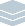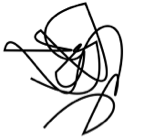Wolfram Archive
Wolfram Programming Lab is a legacy product.
All the same functionality and features, including access to Programming Lab Explorations, are available with Wolfram|One.
Start programming now. »
Try it now »
(no sign-in required)# Random Scribbles in 2D and 3D

Simulate scribbling by joining random points using smooth curves.

Run the code to make a line joining 30 random points. Try more than 30 points:

This draws a line through the points at coordinates {0,0}, {1,1}, and {2,0}:

Graphics[Line[{{0, 0}, {1, 1}, {2, 0}}]]

Turn on axes with AxesTrue in order to see the numeric coordinate positions:

Graphics[Line[{{0, 0}, {1, 1}, {2, 0}}], Axes -> True]

Use RandomReal to draw a line with random coordinates.

This gives a random number between 0 and 1. Each time you run the code, you get a different number:

RandomReal

This gives a pair of numbers between 0 and 1. Each time you run the code, you get a different pair:

RandomReal[1, 2]

This gives four pairs of random numbers:

RandomReal[1, {4, 2}]

Use RandomReal to draw a line with 30 random coordinates:

Graphics[Line[RandomReal[1, {30, 2}]]]

Graphics[Line[RandomReal[1, {30, 2}]]]

Join the points with a Bezier curve instead. Try more than 30 points:

Make a smooth curve by replacing Line with BezierCurve.

Heres a Line through 6 random points (you can ignore SeedRandom for the moment; well explain it later):

SeedRandom; Graphics[Line[RandomReal[1, {6, 2}]]]

Heres a BezierCurve based on the same 6 points:

SeedRandom; Graphics[BezierCurve[RandomReal[1, {6, 2}]]]

Here are the Line and BezierCurve drawn together so you can see how they correspond. The Bezier curve doesnt pass through all of the points, but is guided by them:

Graphics[{ SeedRandom; Line[RandomReal[1, {6, 2}]], Red, SeedRandom; BezierCurve[RandomReal[1, {6, 2}]] }]

Graphics[BezierCurve[RandomReal[1, {30, 2}]]]

Make the curve thicker. Try thicknesses other than 5for example, 10:

AbsoluteThickness specifies how thick to draw lines and curves. The thickness is measured in points, a standard measure used by printers (one point is 1/72 inch):

Graphics[{AbsoluteThickness, BezierCurve[RandomReal[1, {30, 2}]]}]

Graphics[{AbsoluteThickness, BezierCurve[RandomReal[1, {30, 2}]]}]

Make an interactive scribble, with a seed to determine the random choices. Drag the sliders to get different scribbles:

This makes a random scribble with 30 points:

Graphics[{AbsoluteThickness, BezierCurve[RandomReal[1, {30, 2}]]}]

You can make an interactive interface to control the number of points in the scribble using Manipulate.

Wrap the scribble expression with Manipulate[...], replace 30 with the variable length, and specify that length varies from 10 to 200 in steps of 1. Drag the slider to get random scribbles of various lengths:

Manipulate[ Graphics[{AbsoluteThickness, BezierCurve[RandomReal[1, {length, 2}]]}], {length, 10, 200, 1} ]

The scribble jumps around as you drag the slider because RandomReal gives different coordinate positions each time.

If you add SeedRandom to the code, the scribble stays the same as you drag the slider, but gets longer and shorter. Thats because SeedRandom resets the random number generator to the same point each time the scribble is drawn, so that you get the same sequence of random numbers:

Manipulate[ SeedRandom; Graphics[{AbsoluteThickness, BezierCurve[RandomReal[1, {length, 2}]]}], {length, 10, 200, 1} ]

You can give SeedRandom a different number54321 instead of 12345to reset the random number generator to a different point, and get a different scribble:

Manipulate[ SeedRandom; Graphics[{AbsoluteThickness, BezierCurve[RandomReal[1, {length, 2}]]}], {length, 10, 200, 1} ]

You might as well make the seed interactive too, so that you have a control that chooses the scribble:

Manipulate[ SeedRandom[seed]; Graphics[{AbsoluteThickness, BezierCurve[RandomReal[1, {length, 2}]]}], {length, 10, 200, 1}, {seed, 12345, 67890, 1} ]

Manipulate[ SeedRandom[seed]; Graphics[{AbsoluteThickness, BezierCurve[RandomReal[1, {length, 2}]]}], {length, 10, 200, 1}, {seed, 12345, 67890, 1} ]

Draw a random line in 3D. Try more than 30 points:

If you know how to draw scribbles in 2D, its easy to do the same thing in 3D.

Heres a 2D scribble:

Graphics[{AbsoluteThickness, Line[RandomReal[1, {30, 2}]]}]

Replace Graphics with Graphics3D and RandomReal[1,{30,2}] with RandomReal[1,{30,3}] so the line has 3D coordinates instead of 2D. Thats it. You can rotate the scribble with the mouse:

Graphics3D[{AbsoluteThickness, Line[RandomReal[1, {30, 3}]]}]

Graphics3D[{AbsoluteThickness, Line[RandomReal[1, {30, 3}]]}]

Draw a Bezier curve scribble in 3D. Try more than 30 points, or thicknesses other than 5:

Make a smooth 3D curve by replacing Line with BezierCurve:

Graphics3D[{AbsoluteThickness, BezierCurve[RandomReal[1, {30, 3}]]}]

Graphics3D[{AbsoluteThickness, BezierCurve[RandomReal[1, {30, 3}]]}]

Make it interactive. Drag the sliders to get different 3D scribbles. Rotate the scribbles with the mouse:

Manipulate[ SeedRandom[seed]; Graphics3D[{AbsoluteThickness, BezierCurve[RandomReal[1, {length, 3}]]}], {length, 10, 200, 1}, {seed, 12345, 67890, 1} ]

Share ItMake an interactive scribble website:

Deploy the Manipulate to the Wolfram Cloud where anyone with a browser can use it:

CloudDeploy[ Manipulate[ SeedRandom[seed]; Graphics3D[{AbsoluteThickness, BezierCurve[RandomReal[1, {length, 3}]]}], {length, 10, 200, 1}, {seed, 12345, 67890, 1} ], Permissions -> "Public" ]

Click on the link in the output to visit the site.